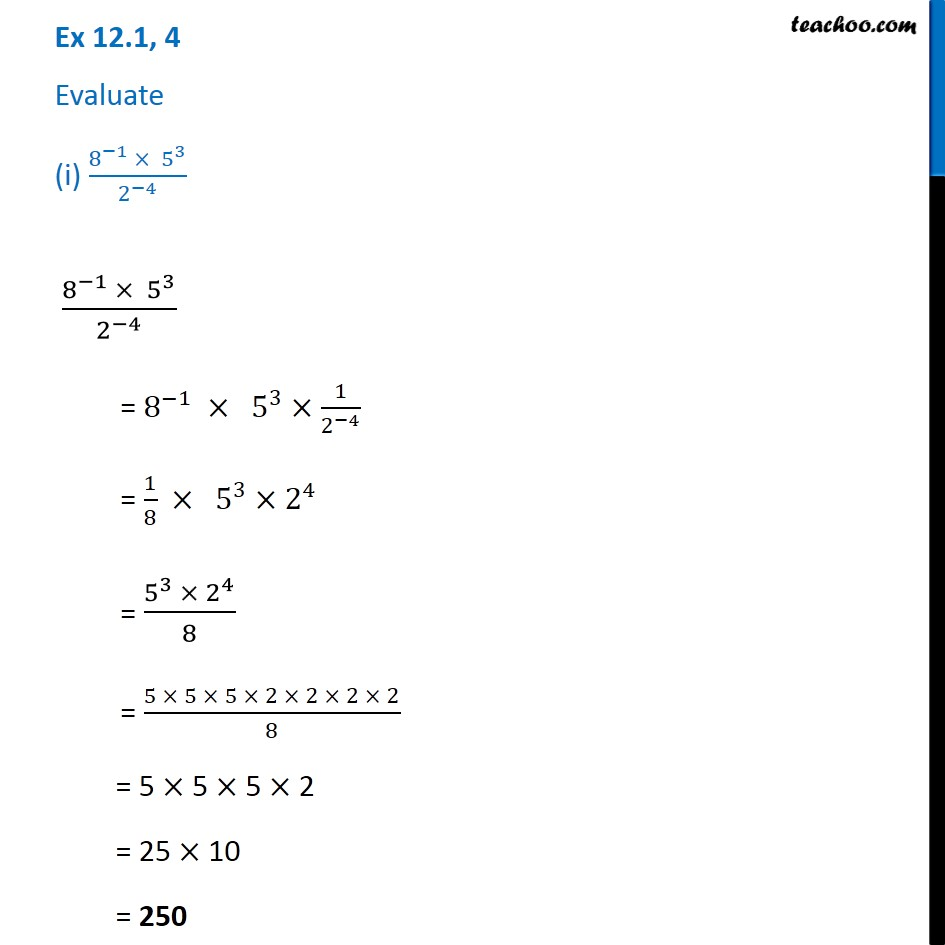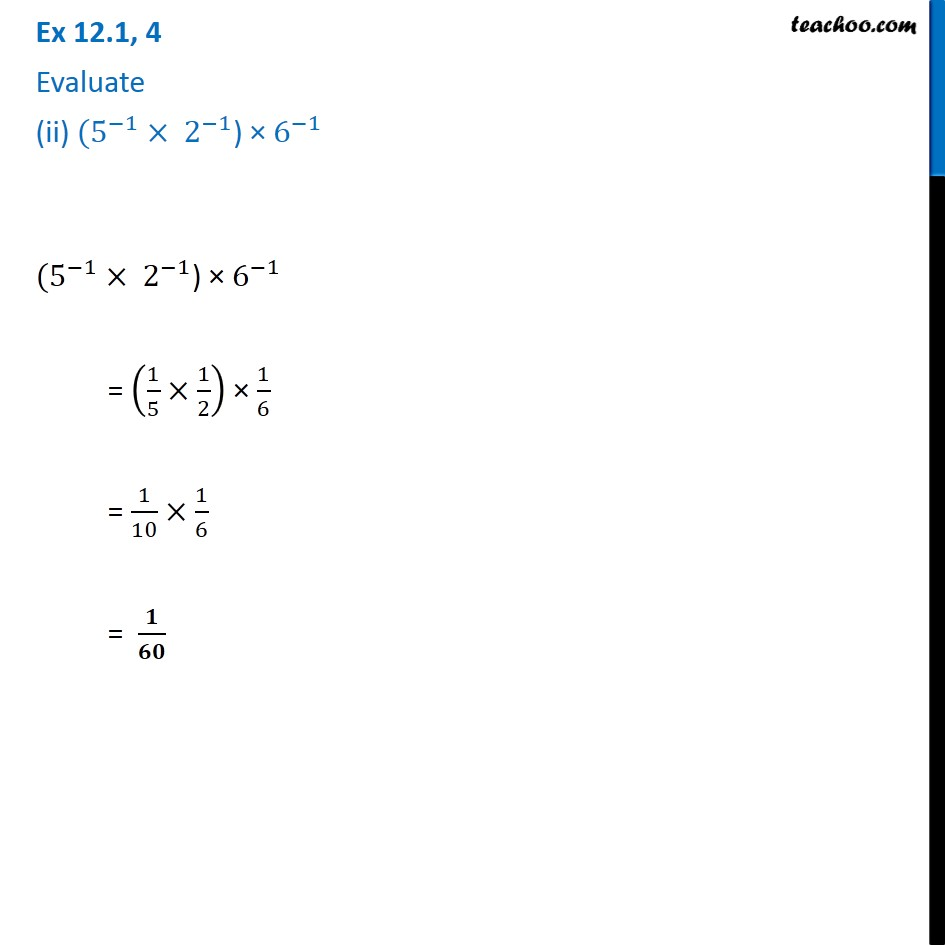1. Chapter 12 Class 8 Exponents and Powers
2. Serial order wise
3. Ex 12.1

Transcript

Ex 12.1, 4 Evaluate (i) (8^(−1) × 5^3)/2^(−4) (8^(−1) × 5^3)/2^(−4) = 8^(−1) × 5^3×1/2^(−4) = 1/8 × 5^3×2^4 = (5^3 × 2^4)/8 = (5 × 5 × 5 × 2 × 2 × 2 × 2)/8 = 5 × 5 × 5 × 2 = 25 × 10 = 250 Ex 12.1, 4 Evaluate (ii) 〖(5〗^(−1) × 2^(−1)) × 6^(−1) 〖(5〗^(−1) × 2^(−1)) × 6^(−1) = (1/5×1/2) × 1/6 = 1/10×1/6 = 𝟏/𝟔𝟎

Ex 12.1